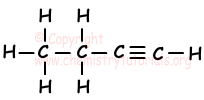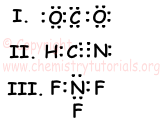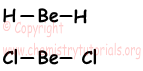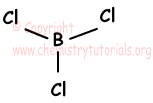## Chemical Bonds Exam1 and Problem Solutions

Chemical Bonds Exam1 and Problem Solutions

1. Find number of σ and π bonds in C4H6 compound.

Solution:

We draw bonds of C4H6;As you can see from the diagram, there are 9 σ and 2 π bonds in C4H6.

2. Which ones of the following statements are exactly true for chemical bonds?

I. While breaking chemical bond, energy is released

II. Two nonmetal atoms share their electrons and form bond

III. Number of electrons lost by metals is equal to group number of it.

Solution:

I. While breaking chemical bonds, we must give energy so I is false.

II. Two nonmetal atoms form bond by electron sharing. II is true.

III. This statement is true for metals in A group. However, it is not true for B group metals. III is false.

3. Which ones of the following Lewi's dot formulas are true? (C:12,, N:14, O:16, H:1)Solution:

Electron configurations of these elements;

1H: 1s1 and H has 1 valence electron

6C: 1s22s22p2 C has 4valence electrons

7N: 1s22s22p3 N has 5 valence electrons

8O: 1s22s22p4 O has 6 valence electrons

9F: 1s22s22p5 N has 7 valence electrons

I. In CO2 molecule, total number of valence electrons must be (4+2.6) 16. However, in electron dot formula we see that there are 20 valence electrons. I is false.

II. In HCN there are 1+4+5=10 valence electrons. Wee see that there are also 10 valence electrons in electron dot formula. II is true.

III. In NF3 molecule there are 5+3.7=26 valence electrons. However, in Lewi's dot formula we see that there are 8 valence electrons.III is false.

4. Which ones of the following statements are true for BeH2, BeCl2 and BCl3? (H:1, Be:4, B:5, Cl:17)

I. BeH2 and BeCl2 has same molecular shapes

II. BCl3 has trigonal planar molecular shape.

III. All of them are nonpolar.

Solution:

I. As you can see from the drawing given below, both BeH2 and BeCl2 has same shape "linear".I is true.II. BCl3 has trigonal planar shape. II is true.III. All of them are nonpolar molecules. III is true.

5. Which one of the following statements is not general properties of metals?

I. They have molecular structures

II. They have many empty orbitals

III. They conduct electric current

IV. They conduct heat

V. They are ductile

Solution:

Metals do not form covalent bonds, thus they do not have molecular structures. I is false. Others are general properties of metals.

Related